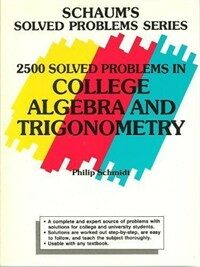> 상세정보

# 상세정보## 2500 solved problems in college algebra and trigonometry (1회 대출)

자료유형
단행본
개인저자
Schmidt, Philip A.
서명 / 저자사항
2500 solved problems in college algebra and trigonometry / by Philip Schmidt.
발행사항
New York :   McGraw-Hill ,   c1991.
형태사항
v, 373 p. : ill. ; 28 cm.
총서사항
Schaum's solved problems series
ISBN
0070553734 :
일반주기
Includes index.
내용주기
Equations and inequalities -- Graphs, relations, and functions -- Polynomial and rational functions -- Systems of equations and inequalities -- Exponential and logarithmic functions -- Trigonometric functions -- Trigonometric equations and inequalities -- Additional topics in trigonometry -- Conic sections -- The complex numbers -- Sequences, series, and probability.
일반주제명
Algebra --Problems, exercises, etc. Trigonometry --Problems, exercises, etc.
비통제주제어
Algebra,,
 000 01321camuuu200301 a 4500 001 000000477148 003 OCoLC 005 19970421112248.0 008 891229s1991 nyua 001 0 eng 010 ▼a 89078522 015 ▼a GB91-27884 020 ▼a 0070553734 : ▼c \$19.95 040 ▼a DLC ▼c DLC ▼d UKM ▼d CNU 049 ▼a ACSL ▼l 421117182 050 0 0 ▼a QA157 ▼b .S247 1991 082 0 0 ▼a 512/.13/076 ▼2 20 090 ▼a 512.13076 ▼b S353t 100 1 ▼a Schmidt, Philip A. 245 1 0 ▼a 2500 solved problems in college algebra and trigonometry / ▼c by Philip Schmidt. 260 ▼a New York : ▼b McGraw-Hill , ▼c c1991. 300 ▼a v, 373 p. : ▼b ill. ; ▼c 28 cm. 440 0 ▼a Schaum's solved problems series 500 ▼a Includes index. 505 1 ▼a Equations and inequalities -- Graphs, relations, and functions -- Polynomial and rational functions -- Systems of equations and inequalities -- Exponential and logarithmic functions -- Trigonometric functions -- Trigonometric equations and inequalities -- Additional topics in trigonometry -- Conic sections -- The complex numbers -- Sequences, series, and probability. 650 0 ▼a Algebra ▼x Problems, exercises, etc. 650 0 ▼a Trigonometry ▼x Problems, exercises, etc. 653 ▼a Algebra 740 0 1 ▼a Twenty-five hundred solved problems in college algebra and trigonometry.

### 소장정보

No. 소장처 청구기호 등록번호 도서상태 반납예정일 예약 서비스
No. 1 소장처 청구기호 512.13076 S353t 등록번호 421117182 도서상태 대출가능 반납예정일 예약 서비스

### 관련분야 신착자료

#### Algebra

Aluffi, Paolo (2021)

김대수 (2022)

민만식 (2022)